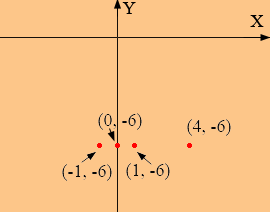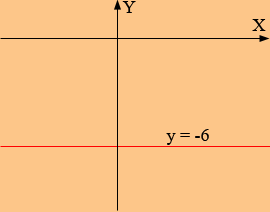SEARCH HOMEMath Central Quandaries & QueriesQuestion from Barb, a student: I was given the problem that says "graph the line y = -6" how do I find all the set points that have a y coordinate of -6? I am so lost lost and have no clue how to start. Can you help me please? thanks.Hi Barb,

Did you plot a few points? I plotted the points that satisfy y = -6 and x = 0, x = 1, x = -1 and x = 4.All these points are 6 units below the X-axis. In fact every point that is 6 units below the X-axis has its Y-value equal to -6. Thus that's the graph of y = -6, all the points in the plane that are 6 units below the X-axis.PennyMath Central is supported by the University of Regina and The Pacific Institute for the Mathematical Sciences.